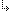- 4.6.1. -4. Standard Units4.6. CompMath - Complex number manipulation Unit4.6.1. CompMath Unit Types

## 4.6.1. CompMath Unit Types

Targets: MS-DOS, OS/2, Win32

CompMath Unit

Following types are defined in the CompMath unit:
```type
CReal = Extended;

type
Complex = record
re, im: CReal
end;

type
TComplex = Complex;```
The Complex type describes a record that stores two fields of type CReal (see above), one that represents the real part of a complex number and one that represents the imaginary part.

- 4.6.1. -